# JP4066484B2 - Image processing apparatus, image processing method, and camera - Google Patents

## Info

Publication number
JP4066484B2
JP4066484B2 JP33674497A JP33674497A JP4066484B2 JP 4066484 B2 JP4066484 B2 JP 4066484B2 JP 33674497 A JP33674497 A JP 33674497A JP 33674497 A JP33674497 A JP 33674497A JP 4066484 B2 JP4066484 B2 JP 4066484B2
Authority
JP
Japan
Prior art keywords
interpolation
directions
correlation
pixel
coefficient
Prior art date
Legal status (The legal status is an assumption and is not a legal conclusion. Google has not performed a legal analysis and makes no representation as to the accuracy of the status listed.)
Application number
JP33674497A
Other languages
Japanese (ja)
Other versions
JPH11177994A (en
Inventor

Original Assignee
ソニー株式会社
Priority date (The priority date is an assumption and is not a legal conclusion. Google has not performed a legal analysis and makes no representation as to the accuracy of the date listed.)
Filing date
Publication date
Application filed by ソニー株式会社 filed Critical ソニー株式会社
Priority to JP33674497A priority Critical patent/JP4066484B2/en
Publication of JPH11177994A publication Critical patent/JPH11177994A/en
Application granted granted Critical
Publication of JP4066484B2 publication Critical patent/JP4066484B2/en
Anticipated expiration legal-status Critical
Application status is Expired - Lifetime legal-status Critical

## Images

•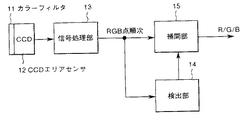•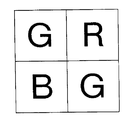•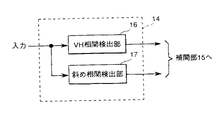•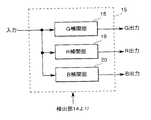•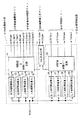•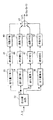•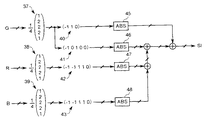•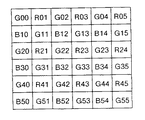•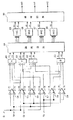•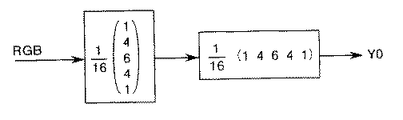••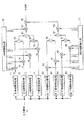•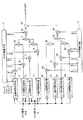•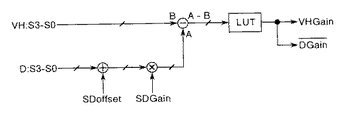•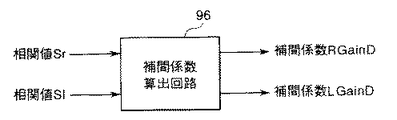•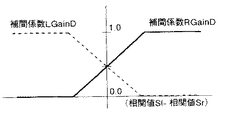•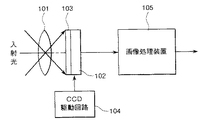## Classifications

• GPHYSICS
• G06COMPUTING; CALCULATING; COUNTING
• G06TIMAGE DATA PROCESSING OR GENERATION, IN GENERAL
• G06T3/00Geometric image transformation in the plane of the image
• G06T3/40Scaling the whole image or part thereof
• G06T3/4007Interpolation-based scaling, e.g. bilinear interpolation
• GPHYSICS
• G06COMPUTING; CALCULATING; COUNTING
• G06TIMAGE DATA PROCESSING OR GENERATION, IN GENERAL
• G06T3/00Geometric image transformation in the plane of the image
• G06T3/40Scaling the whole image or part thereof
• G06T3/4015Demosaicing, e.g. colour filter array [CFA], Bayer pattern
• HELECTRICITY
• H04ELECTRIC COMMUNICATION TECHNIQUE
• H04NPICTORIAL COMMUNICATION, e.g. TELEVISION
• H04N9/00Details of colour television systems
• H04N9/04Picture signal generators
• H04N9/045Picture signal generators using solid-state devices
• HELECTRICITY
• H04ELECTRIC COMMUNICATION TECHNIQUE
• H04NPICTORIAL COMMUNICATION, e.g. TELEVISION
• H04N2209/00Details of colour television systems
• H04N2209/04Picture signal generators
• H04N2209/041Picture signal generators using solid-state devices
• H04N2209/042Picture signal generators using solid-state devices having a single pick-up sensor
• H04N2209/045Picture signal generators using solid-state devices having a single pick-up sensor using mosaic colour filter
• H04N2209/046Colour interpolation to calculate the missing colour values

## Description


BACKGROUND OF THE INVENTION
The present invention relates to an image processing apparatus, an image processing method, and a camera, and more particularly to an image processing apparatus and an image processing method for processing an output signal of a solid-state imaging device having a color filter of a predetermined color arrangement on a light receiving surface, and It relates to the camera used.

[Prior art]
In an image processing apparatus that processes an output signal of a solid-state imaging device having a color filter of, for example, a primary color Bayer array on the light receiving surface, each color is scattered on the color filter so that the resolution is not impaired. Interpolation processing is performed to achieve this. In this type of image processing apparatus, conventionally, correlation values in only two directions, such as two opposite directions in the horizontal direction (left and right direction) or two opposite directions in the vertical direction (up and down direction), are calculated and used as the basis. Interpolation processing was performed. In other words, whether horizontal stripes or vertical stripes are determined from the pixel information of, for example, a 5 × 5 pixel area around the pixel to be interpolated, and in the case of horizontal stripes, interpolation is performed using the signals of pixels on both sides in the horizontal direction of the pixel to be interpolated. In the case of vertical stripes, interpolation is performed using the signals of pixels on both sides in the vertical direction.

[Problems to be solved by the invention]
However, in the conventional image processing apparatus that performs interpolation by detecting the correlation in only two directions, it is effective for the edge portion in the horizontal direction or the vertical direction, but one pixel is also used for the oblique edge portion or the edge in the horizontal / vertical direction. In the case of a line segment of about the width, it is not possible to detect an appropriate edge at the intersection of the horizontal edge and the vertical edge, or in the bent part of the line segment, and in the case of an oblique line, is the horizontal correlation strong? Whether or not the vertical correlation is strong cannot be determined, and the process of averaging the horizontal edge portion process and the vertical edge portion process (average value interpolation) tends to be performed.

For this reason, in the conventional image processing apparatus, in the portion shown in the above example, there is a problem that the image is blurred with no resolution, or the line is interrupted in a portion that should not be interrupted. become.

The present invention has been made in view of the above problems, and an object of the present invention is to provide an image processing apparatus capable of performing satisfactory interpolation processing even in a bent line or a corner portion bent at a right angle. And providing an image processing method.

[Means for Solving the Problems]
An image processing apparatus according to the present invention includes a solid-state imaging device having a color filter having a predetermined color arrangement on a light receiving surface.Is obtained by passing a low-pass filter in the direction of interpolation with respect to the pixel to be interpolated when color correlation processing is performed by detecting the correlation between the pixel to be interpolated and its surrounding pixels. Interpolation processing is performed by multiplying the interpolation data by the interpolation coefficient and adding the multiplication results.An image processing apparatus, which forms an angle that is an integral multiple of 90 ° with respect to a pixel to be interpolated (hereinafter referred to as an interpolated pixel).Pixel information corresponding to 4 directionsA correlation value calculation circuit for calculating a correlation value for the four directions based on the correlation value, a coefficient determination circuit for determining an interpolation coefficient based on the correlation values for the four directions calculated by the correlation value calculation circuit, and the coefficient determination circuit Using the interpolation coefficient determined in step 4 aboveCorresponding pixel informationInterpolation processing circuit that performs interpolation processing based onThe coefficient determination circuit determines a correlation mode in accordance with the magnitude relationship of each correlation value with respect to the four directions, and changes the calculation method of the interpolation coefficient according to the correlation mode..

The image processing method according to the present invention also includes a solid-state imaging device having a color filter having a predetermined color arrangement on a light receiving surface.Is obtained by passing a low-pass filter in the direction of interpolation with respect to the pixel to be interpolated when color correlation processing is performed by detecting the correlation between the pixel to be interpolated and its surrounding pixels. Interpolation processing is performed by multiplying the interpolation data by the interpolation coefficient and adding the multiplication results.An image processing method that forms an angle that is an integral multiple of 90 ° on the left, right, and top and bottom with respect to an interpolated pixelPixel information corresponding to 4 directionsCalculate correlation values for the four directions based onCalculation step to,thisIn the calculation stepInterpolation coefficient is determined based on the calculated correlation values for the four directionsWith a decision step to,thisIn the decision stepBased on the determined interpolation coefficient in the above four directionsCorresponding pixel informationPerform interpolation processing based onAnd a processing step. In the determining step, a correlation mode is determined according to the magnitude relationship of the correlation values with respect to the four directions, and the calculation method of the interpolation coefficient is changed according to the correlation mode.

In the image processing apparatus having the above configuration and its processing method, first, a characteristic value for a total of four directions of the interpolated pixel, that is, left and right (horizontal direction) and up and down (vertical direction), that is, a correlation value indicating a degree (degree) of correlation is calculated. . Next, based on the correlation values for the four directions, an interpolation coefficient used at the time of interpolation is determined.In determining the interpolation coefficient, the correlation mode is determined according to the magnitude relationship of the correlation values with respect to the four directions, and the calculation method of the interpolation coefficient is changed depending on the correlation mode.And used to calculate the correlation valuePixel information corresponding to 4 directionsInterpolation processing is performed by multiplying the interpolation coefficients and adding them.

DETAILED DESCRIPTION OF THE INVENTION
Hereinafter, embodiments of the present invention will be described in detail with reference to the drawings. FIG. 1 is a block diagram showing a basic configuration of an image processing apparatus according to the present invention. Here, the color solid-state imaging device to be processed by the image processing apparatus uses, for example, the color filter 11 of the primary color Bayer array of R (red) G (green) B (blue) shown in FIG. This is a single plate type solid-state imaging device 12.

Note that the color arrangement is not limited to the primary color Bayer arrangement, and the color filter is not limited to the RGB primary color arrangement, and even in the case of other primary color arrangements, a color arrangement using complementary colors ( For example, the case of Ye / Cy / Mg / G) can be similarly applied. Further, as the solid-state imaging device 12, a so-called all-pixel readout type CCD (Charge Coupled Device) solid-state imaging device (hereinafter referred to as a CCD area sensor) that reads out signal charges of all the pixels independently is used. The present invention can also be applied to a CCD solid-state imaging device that is not a pixel readout method.

The RGB point sequential data output from the CCD area sensor 12 is subjected to signal processing such as black level clamping and white balance in the signal processing unit 13 and then supplied to the detection unit 14 and the interpolation unit 15. The detection unit 14 detects an optimal interpolation method from the input RGB point sequential data and sends the interpolation information to the interpolation unit 15. The interpolation unit 15 performs an interpolation process on the RGB point sequential data based on the interpolation information input from the detection unit 14 and outputs the result.

As shown in FIG. 3, the detection unit 14 has four directions that form an angle that is an integral multiple of 90 ° with respect to the interpolated pixel, that is, the vertical (V) direction and the opposite two directions and the horizontal (H) direction. A VH correlation detection unit 16 that detects the degree of correlation in a total of four directions in two opposite directions, and an oblique direction of upper right, upper left, lower left, and lower right with respect to the interpolation pixel, that is, an angle of 45 ° with respect to the above four directions. The oblique correlation detection unit 17 detects the degree of correlation in the four directions formed.

In this example, a configuration that detects the degree of correlation in a total of eight directions in four oblique directions in addition to the four directions in the upper, lower, left, and right directions is used as an example. It may be. However, in the following description, the case of 8 directions will be described as an example.

On the other hand, as illustrated in FIG. 4, the interpolation unit 15 performs G interpolation on the G pixel information based on the interpolation information provided from the detection unit 14, and the R pixel information. The configuration includes an R interpolation unit 19 that performs interpolation processing and a B interpolation unit 20 that performs interpolation processing on B pixel information.

FIG. 5 is a block diagram illustrating an example of a specific configuration of the VH correlation detection unit 16 and the diagonal correlation detection unit 17.

The VH correlation detection unit 16 calculates the correlation value indicating the degree of correlation based on the pixel information of the pixel on the right side of the interpolation pixel, and correlates based on the pixel information of the pixel on the left side of the interpolation pixel. A left correlation value calculation circuit 22 that calculates a value, an upper correlation value calculation circuit 23 that calculates a correlation value based on pixel information of an upper pixel of the interpolation pixel, and a pixel information of a lower pixel of the interpolation pixel A lower correlation value calculation circuit 24 that calculates correlation values, and a correlation value → interpolation gain conversion circuit 25 that converts each correlation value calculated by the correlation value calculation circuits 21 to 24 into an interpolation gain and outputs the result. Yes.

In the VH correlation detection unit 16 having the above-described configuration, the correlation value → interpolation gain conversion circuit 25 uses the horizontal and vertical interpolation gains RGain, LGain, TGain, and BGain and the horizontal and vertical RB interpolation gains RGainD, LGainD, TGainD, and BGainD as interpolation coefficients. Is output as The horizontal and vertical RB interpolation gains RGainD, LGainD, TGainD, and BGainD will be described in detail later.

The oblique correlation detection unit 17 calculates the correlation value based on the pixel information of the pixel on the upper right side of the interpolation pixel, and calculates the correlation value based on the pixel information of the pixel on the upper left side of the interpolation pixel. The upper left correlation value calculation circuit 27 to calculate, the lower left correlation value calculation circuit 28 to calculate the correlation value based on the pixel information of the lower left pixel of the interpolation pixel, and the pixel information of the lower right pixel of the interpolation pixel Lower right side correlation value calculation circuit 29 for calculating a correlation value based on this, and each correlation value calculated by these correlation value calculation circuits 26 to 29 is converted into an interpolation gain, and diagonal interpolation gains D1 Gain to D4 Gain are output as interpolation coefficients. Correlation value → interpolation gain conversion circuit 30.

Further, since the four horizontal and vertical directions and the four oblique directions are not orthogonal to each other, the VH-diagonal comparison circuit 31 compares the horizontal and vertical correlation values with the diagonal correlation values, thereby obtaining the diagonal interpolation correction gains VHGain and DGain. Is calculated as an interpolation coefficient. A method of calculating the diagonal interpolation correction gains VHGain and DGain will be described in detail later.

FIG. 6 is a block diagram illustrating an example of a specific configuration of, for example, the left correlation value calculation circuit 22 in the VH correlation detection unit 16. The right side correlation value calculation circuit 21, the upper side correlation value calculation circuit 23, and the lower side correlation value calculation circuit 24 have the same circuit configuration.

In the left correlation value calculation circuit 22, the input RGB point sequential signal is separated into an R signal / G signal / B signal corresponding to each color of R pixel / G pixel / B pixel and output by a color separation circuit 32. Is done. The G signal is supplied to the change amount 1, 2 calculation circuits 33, 34, respectively. The R signal is supplied to the change amount calculation circuit 35. The B signal is supplied to the change amount calculation circuit 36.

Here, only the G signal is input to the two different change amount 1, 2 calculation circuits 33, 34, as can be seen from the RGB primary color Bayer array in FIG. 2, G is compared to R / B. This is because the number of samplings in the horizontal direction and the vertical direction is double, and in G, the amount of change 1 between adjacent vertical lines and the amount of change 2 between one vertical line are calculated. Here, an example was taken between adjacent vertical lines or between one vertical line, but it may be between two or more vertical lines.

As shown in the conceptual diagram of FIG. 7, the change amount 1, 2 calculation circuits 33, 34 and the change amount calculation circuits 35, 36 are LPFs (1, 2, 2) oriented in a direction orthogonal to the direction in which the change amount is calculated. 2, 2, 1) After passing through 37, 38, 39, the change amount is calculated by passing through BPFs 40, 41, 42, 43 for calculation. Here, the calculation BPFs 40, 41, 42, and 43 use 1/2 correlation (1, -1) and 1/4 correlation (1, 0, -1) for G, and for R / B. 1/4 correlation (1, 1, -1, -1) is used.

The change amounts calculated by the change amount calculation circuits 33, 34, 35, and 36 are converted into absolute values by the absolute value conversion circuits 45, 46, 47, and 48 and then sent to the coefficient addition circuits 49, 50, 51, and 52. Supplied. The coefficient addition circuits 49, 50, 51, and 52 multiply each change amount converted into an absolute value by the absolute value conversion circuits 45, 46, 47, and 48 by multiplying a separately set coefficient. The outputs of the coefficient adding circuits 49, 50, 51, and 52 are added together by the adder circuit 53, and then output as upper, lower, left, and right correlation values St, Sb, S1, and Sr. It should be noted that RB is added so that the contribution to the correlation value of RB is lower than that of G.

Here, the reason for multiplying the coefficients in the coefficient adding circuits 49, 50, 51 and 52 is that each color of R / G / B is 0.30R + 0.59G + 0. This is to match the human visual characteristics as calculated as 11B. However, in order to reduce the calculation amount and the gate scale, it is simple that R: G: B has a ratio of 3: 6: 1, a ratio of 1: 2: 1, or a ratio of 2: 4: 1. It is better to set the coefficient.

Next, a method of calculating the amount of change in the left direction by the amount of change calculation circuits 33 to 36 in the left correlation value calculation circuit 22 having the above configuration will be described with reference to the primary color Bayer array diagram of FIG. Here, a method of calculating the amount of change in the left direction will be described, but the other directions (right / up / down directions) are exactly the same except that the subscripts are changed.

First, for G, the amount of change between adjacent vertical lines is calculated from equation (1), and the amount of change between one vertical line is calculated from equation (2). In addition, in Formula (1) / Formula (2), the formula at the time of detecting the correlation with respect to the pixel G22 is shown. On the other hand, in the R signal / B signal, the same color signal exists only every other line, and therefore, the amount of change between the vertical lines of one line is calculated by the equations (3) / (4). Further, for the pixel G33, the subscript indicating horizontal / vertical is increased by 1, and the calculation method is switched between the R signal and the B signal.

[Expression 1]

In the VH correlation detection unit 16 shown in FIG. 5, the correlation value → correlation gain conversion circuit 25, which is a circuit for determining the correlation coefficient, is calculated by the correlation value calculation circuits 21 to 24 in determining the correlation coefficient. The correlation mode is determined based on the correlation values Sr, S1, St, and Sb in the four directions. The reason why the correlation mode is introduced is that it is difficult to determine the interpolation coefficient for the correlation order when the correlation values are simply ranked.

For example, when the four correlation values are almost the same (100, 101, 102, 103) and when they are completely different (0, 10, 100, 500), the interpolation coefficient calculation results should be different. Therefore, a correlation mode indicating the distribution of correlation coefficients is introduced separately from the correlation order determination. The correlation mode represents the distribution of the four correlation values Sr, S1, St, and Sb. As shown in Table 1, the correlation mode includes eight modes from 0 to 7.

[Table 1]

FIG. 9 is a block diagram showing an example of a specific configuration of a correlation mode determination circuit for determining a correlation mode.

In FIG. 9, six comparators 54 to 59 for comparing four correlation values Sr, St, Sl, and Sb, respectively, are provided. The correlation value Sr in the right direction becomes one comparison input A of each of the comparators 54, 55, and 57. The upward correlation value St becomes the other comparison input B of the comparator 54 and also becomes one comparison input A of each of the comparators 56 and 58. The correlation value S1 in the left direction becomes the other comparison input B of each of the comparators 55 and 56 and also becomes one comparison input A of the comparator 59. The downward correlation value Sb becomes the other comparison input B of each of the comparators 57, 58 and 59.

Here, the comparators 54 to 59 compare the two comparison inputs A and B, and output a comparison result of three stages of “>” (large), “<” (small), and “=” (equivalent). It is the composition to do. That is, in addition to the two comparison results of A> B and A <B, if the absolute value of the difference between the two correlation values to be compared is smaller than a certain reference value TH, it is assumed that “=”. As the reference value TH, an average level or a weighted average level of pixels in the vicinity of the interpolation pixel, for example, a value obtained by multiplying the luminance Y0 in the vicinity of the interpolation pixel by a coefficient. FIG. 10 is a conceptual diagram of detection of the luminance Y0.

At the subsequent stage of the comparators 54 to 59, four 1-bit full adders 60 to 63 corresponding to right, upper, left, and lower are provided. The full adder 60 uses the comparison result A> B of the comparator 54 as one addition input X, the comparison result A> B of the comparator 55 as the other addition input Y, and the comparison result A> B of the comparator 57 as a carry input C. To do. The full adder 61 uses the comparison result A <B of the comparator 54 as one addition input X, the comparison result A> B of the comparator 56 as the other addition input Y, and the comparison result A> B of the comparator 58 as a carry input C. To do.

The full adder 62 uses the comparison result A <B of the comparator 55 as one addition input X, the comparison result A <B of the comparator 56 as the other addition input Y, and the comparison result A> B of the comparator 59 as a carry input C. To do. The full adder 63 uses the comparison result A <B of the comparator 57 as one addition input X, the comparison result A <B of the comparator 58 as the other addition input Y, and the comparison result A <B of the comparator 59 as a carry input C. To do. The full adders 60 to 63 determine their rank based on the two addition inputs X and Y and the carry input C, and output the determination results S0 and S1.


In the correlation mode determination circuit configured as described above, the four correlation values Sr, S1, St, and Sb are respectively compared to obtain three levels of comparison results (“>”, “<”, “=”). Based on the order starting from 0 in ascending order. In this case, 2 to 4 directions may have the same order. Based on this distribution of rank, the correlation mode is determined according to Table 1. In the case of Modes 1, 2, 5, and 6, the distribution may be different from the table. For example, in Mode 1, rank 0 may be in three directions, and the remaining rank in one direction may be any one of 1, 2, and 3.

Subsequently, ranking is performed from the correlation order. Here, the rank and the correlation order are different for convenience. The rank is an order with respect to directions in the same correlation order. In the case of the same correlation order, the ordering method is clockwise from “right”. For example, if the top, bottom, left, and right have the same correlation rank, the ranks of right, top, left, and bottom are 0, 1, 2, and 3, respectively.

As described above, in the correlation value → correlation gain conversion circuit 25, the correlation mode and rank are determined when calculating the correlation coefficient, and the interpolation coefficient of each rank is calculated by this combination. Interpolation coefficients, that is, horizontal / vertical interpolation gains RGain, LGain, TGain, BGain and horizontal / vertical RG interpolation gains RGainD, LGainD, TGainD, BGainD can be determined.

Here, in calculating the interpolation coefficient, the correlation value → correlation gain conversion circuit 25 adopts a configuration in which the interpolation coefficient calculation method is changed according to the determined correlation mode. That is, among the four correlation values Sr, S1, St, and Sb in the four horizontal and vertical directions, S0, S1, S2, and S3 are set in ascending order, and a lookup table (LUT) is set for each mode based on these correlation values. ) To obtain a variable α (0 ≦ α ≦ 1/4) and calculate an interpolation coefficient using this variable α.

That is, as shown in FIG. 11, four correlation values S0 to S3 are calculated in the arithmetic circuit 70A to obtain three calculation outputs S3-S0, S2-S0, and S1-S0. The three operation outputs S3-S0, S2-S0, and S1-S0 are selected by the selection circuit 70B corresponding to each mode determined by the 3-bit mode signals m0 to m2, and are given to the lookup table 70C. To do.

In the look-up table 70C, α = 0 in mode 0, variable α corresponding to the value of S3-S0 in mode 1, and variable α corresponding to the value of S2-S0 in mode 2 or mode 3, Thereafter, the variable α corresponding to the value of S1-S0 is set. Then, using this variable α, an interpolation coefficient is calculated as shown in Table 2. Note that the value of the variable α can be arbitrarily set by making the table value of the lookup table 70C changeable from the outside.

[Table 2]

Although the correlation detection in the four horizontal and vertical directions has been described above, for the correlation detection in the four diagonal directions, the absolute value of the modulation component in the diagonal direction is calculated for each RGB signal after color separation, and R: G : B = 1: 4: 1 is added to obtain a relative value. Note that the upper right, upper left, lower left, and lower right are defined as the D1, D2, D3, and D4 directions, respectively. Then, in the correlation value → interpolation gain conversion circuit 30 shown in FIG. 5, the interpolation gains D1Gain, D2Gain, D3Gain, and D4Gain in the four oblique directions are calculated as interpolation coefficients in the same manner as in the four horizontal and vertical directions.

Next, an example of a specific configuration of each of the G, R, and B interpolation units 18, 19, and 20 in the interpolation unit 15 of FIG. 4 is shown in FIGS. 12 shows the configuration of the G interpolation unit 18, and FIG. 13 shows the configuration of the R and B interpolation units 19 and 20, respectively.

First, in FIG. 12, the color-separated G data is supplied to the correlation processing circuits 71, 72, 73, 74 in the four horizontal and vertical directions, that is, the right side, the left side, the upper side, and the lower side, respectively. In the circuits 71, 72, 73, 74, interpolation data Gr, Gl, Gt, Gb in four directions are generated. These interpolation data Gr, Gl, Gt, and Gb are generated by passing the LPF in the interpolation direction. Actually, two LPFs for high luminance and low luminance are provided, and the outputs are multiplied by a predetermined gain and added to generate interpolation data Gr, Gl, Gt, Gb.

The interpolation data Gr, Gl, Gt, and Gb are respectively multiplied by the interpolation coefficients determined by the VH correlation detection unit 16 shown in FIG. 5 in the multipliers 75, 76, 77, and 78, that is, horizontal and vertical interpolation gains RGain, LGain, and TGain. , BGain, respectively. Then, addition is performed by the adders 79 to 81 to perform G interpolation processing. The G image data after the interpolation processing is expressed by equation (5).
G = Gr x RGain + Gl x LGain
+ Gt × TGain + Gb × BGain (5)

Similarly, in the four oblique directions, interpolation data in each direction of D1 / D2 / D3 / D4 is generated in the correlation processing circuits 82, 83, 84, 85 on the upper right side, upper left side, lower left side, and lower right side. In the multipliers 86, 87, 88, and 89, the diagonal interpolation gains D1Gain, D2Gain, D3Gain, and D4Gain determined by the diagonal correlation detector 17 shown in FIG. An interpolation process is performed by adding them.

Further, G image data obtained by horizontal / vertical four-way correlation detection / interpolation and G image data obtained by oblique four-way correlation detection / interpolation are added by changing the mixing ratio according to the situation as shown in the equation (6). To do. The adjustment of the mixing ratio is performed by diagonal interpolation correction gains VHGain and DGain calculated by the VH-diagonal comparison circuit 31 shown in FIG.
G = Gvh × VHGain + Gd × DGain (6)

Here, since the horizontal / vertical direction and the diagonal direction are not orthogonal, the difference between the maximum value S3 and the minimum value S0 (S3−S0) of the horizontal / vertical and diagonal correlation values is compared. Addition is performed so that the interpolation coefficient is larger than the smaller interpolation coefficient. This is because the difference between the maximum value and the minimum value of the correlation value becomes large in the case of an edge that is parallel or orthogonal to the detection direction. However, when the horizontal / vertical and diagonal are compared, an offset is given to the horizontal / vertical calculation value, horizontal / vertical interpolation is performed as a standard, and the diagonal interpolation coefficient is increased only when it is suitable for diagonal interpolation.

When comparing the difference (S3−S0) between the maximum value S3 and the minimum value S0 of the horizontal and vertical correlation values, as shown in the conceptual diagram of FIG. 14, the diagonal difference (S3−S0) is calculated. A value A obtained by adding (or subtracting) a predetermined constant SDoffset to the coefficient SDGain is compared with a horizontal / vertical difference B (S3−S0), and the value A is obliquely determined based on the difference (A−B). The interpolation correction gains VHGain and DGain are set.

That is, in FIG. 12, the multiplier 93 multiplies the output data of the adder 81 by the diagonal interpolation correction gain VHGain, and the multiplier 94 multiplies the output data of the adder 92 by the interpolation correction gain DGain. Each output data of the multipliers 93 and 94 is added by an adder 95, whereby G image data obtained by horizontal / vertical four-way correlation detection / interpolation and G image data obtained by oblique four-way correlation detection / interpolation are predetermined. Mix (add) at a rate.

Although the configuration and operation of the G interpolation unit 18 have been described above, the configurations of the R and B interpolation units 19 and 20 shown in FIG. 13 are basically the same as the configuration of the G interpolation unit 18 shown in FIG. . Therefore, in FIG. 13, the same parts as those in FIG. However, the method of generating interpolation data in each of the correlation processing circuits 71 ′, 72 ′, 73 ′, 74 ′ on the right side, the left side, the upper side, and the lower side is more complicated than the case of G. There are two reasons for this, to perform correlation detection for RB interpolation and to add a G / 2 component to RB.

Therefore, the correlation detection for RG interpolation is performed separately for horizontal (right, left) interpolation data and vertical (up, down) interpolation data. Specifically, when the R signal / B signal is interpolated from the R pixel and B pixel signals, the above-described horizontal / vertical RB interpolation gains RGainD, LGainD, TGainD, and BGainD are calculated as interpolation coefficients dedicated to R / B. And use. The R signal interpolation processing will be described below as an example, but the same applies to the B signal interpolation processing.

In the color filter 11 of the RGB primary color Bayer array, as is apparent from FIG. 2 showing the color array, G is the spatial sampling number in the horizontal direction or the vertical direction and the spatial sampling number by all pixels of the CCD area sensor 12. On the other hand, in the R / B, the spatial sampling number in the horizontal direction or the vertical direction is smaller than the spatial sampling number by all the pixels of the CCD area sensor 12.

In the primary color Bayer array shown in FIG. 8, when the pixel to be interpolated is the R21 pixel, no special processing is required, and the R21 signal is used as it is as the R signal. When the pixel to be interpolated is G22, the left interpolation uses the R21 pixel, and the right interpolation uses the R23 pixel. However, in the case of the upper interpolation and the lower interpolation, the interpolation coefficient calculation circuit 96 shown in FIG. 15 is used. Like that. The interpolation coefficient calculation circuit 96 constitutes a part of the correlation value → correlation gain conversion circuit 25 in the VH correlation detection unit 16 of FIG.

That is, the VH correlation detection unit 16 inputs the right correlation value Sr calculated by the right correlation value calculation circuit 21 and the left correlation value S1 calculated by the left correlation value calculation circuit 22 to the interpolation coefficient calculation circuit 96, and calculates the interpolation coefficient. The circuit 96 calculates the right interpolation coefficient RGainD and the left interpolation coefficient LGainD. An example of the characteristic of the interpolation coefficient calculation circuit 96 is shown in FIG. In the characteristics shown in FIG. 16, the interpolation coefficient is shown in the range of 0.0 to 1.0. However, any number of interpolation coefficients may be used, and the interpolation coefficient and the R signal are actually multiplied. Sometimes, as a result, there is no problem if it falls within the range of 0.0 to 1.0.

When the right interpolation coefficient RGainD and left interpolation coefficient LGainD calculated in this way are input to the right correlation processing circuit 71 'and the left correlation processing circuit 72' of FIG. 13, these correlation processing circuits 71 ', At 72 ′, a predetermined calculation process is performed, and upper interpolation data TData and lower interpolation value BData are calculated. As an arithmetic expression in the correlation processing circuits 71 ′ and 72 ′,
TData = R01 × LGainD + R03 × RGainD
BData = R41 × LGainD + R43 × RGainD

Or
TData = {(R01 + R21) × LGainD
+ (R03 + R23) × RGainD} / 2
BData = {(R21 + R41) × LGainD
+ (R23 + R43) × RGainD} / 2
And so on.

In the case of the pixel G33 to be interpolated, the upper interpolation coefficient TGainD / lower interpolation coefficient BGainD is calculated from the upper correlation value St / lower correlation value Sb by the same processing method as described above. The right interpolation data RData / left interpolation data LData is calculated by the equation.
LData = R21 × TGainD + R41 × BGainD
RData = R25 × TGainD + R45 × BGainD

Further, when the pixel to be interpolated is B32, the upper side correlation value St / lower side correlation value Sb is converted into the upper side interpolation coefficient TGainD / lower side interpolation coefficient BGainD, and the right side correlation value Sr / left side correlation value S1 is converted into the right side interpolation coefficient RGainD /. The left interpolation coefficient LGainD is calculated, and the upper interpolation data TData / lower interpolation data BData / left interpolation data LData / right interpolation data RData are calculated using the following arithmetic expressions.
TData = R21 × LGainD + R23 × RGainD
BData = R41 × LGainD + R43 × RGainD
LData = R21 × TGainD + R41 × BGainD
RData = R23 × TGainD + R43 × BGainD

As described above, the correlation detection for RG interpolation is performed separately for the interpolation data in the horizontal direction (right and left) and the interpolation data in the vertical direction (up and down). For the interpolation coefficients LGainD and RGainD for the interpolation data in the horizontal direction, the right correlation value Sr and the left correlation value S1 are compared (S1−Sr). The vertical correlation data interpolation coefficients TGainD and BGainD are calculated by comparing the upper correlation value St and the lower correlation value Sb (Sb-St).

Then, after calculating the upper interpolation data TData / lower interpolation data BData / left interpolation data LData / right interpolation data RData based on these interpolation coefficients TGainD / BGainD / LGainD / RGainD, the VH correlation detector 16 in FIG. Multiply and add the calculated horizontal / vertical interpolation coefficients TGain / BGain / LGain / RGain based on the four-way correlation detection to generate an R signal.

Here, a case where the upper interpolation data TData for R is generated will be considered as an example. Since R has information only every other pixel in both the horizontal and vertical directions in the original image, when the pixel to be interpolated is an R pixel, the data of that pixel is used, and in the case of a Gb pixel, the upper side is an R pixel. The upper R pixel data is used as upper interpolation data.

However, in the case of the B pixel or the Gr pixel, there is no R information on the vertical line, so the upper interpolation data TData is generated based on the R pixel of the left and right vertical lines and the 1/2 modulation component of G. Specifically, the R pixel on the right vertical line and the horizontal half G modulation component are multiplied by RGainD as a coefficient, and the R pixel on the left vertical line and the horizontal left G 1 / The upper interpolation data TData is generated by multiplying the sum of the two modulation components by multiplying LGainD as a coefficient.

By the way, in the primary color Bayer array shown in FIG. 8, when the R signal corresponding to the pixel of G22 is interpolated, there is no problem because both sides are R and R is sparse with respect to the horizontal direction interpolation. Even if interpolation is performed, only the G signal or the B signal exists in the same vertical line as in G02, B12, G22, B32, and G42. For this reason, it is necessary to use the R signal of the adjacent vertical line.

However, when a vertical edge whose signal level changes rapidly in the horizontal direction is imaged, the edge may be between the vertical line of G22 and the adjacent vertical line of R23, for example. In this case, the level of the R signal at the position of G22 should be almost equal to that of R01 / R21 / R41 and the like, but since the level of the R signal such as R03 / R23 / R43 is used, what is the original signal level? Unlikely, there is a concern that the edge will be colored.

However, in the primary color Bayer array signal processing, as described above, when the R signal / B signal is interpolated from the R pixel and B pixel signals, horizontal interpolation is performed based on the right correlation value Sr and the left correlation value S1. The interpolation coefficients LGainD and RGainD for data are used by calculating and using the interpolation coefficients TGainD and BGainD for the interpolation data in the vertical direction based on the upper correlation value St and the lower correlation value Sb. The resolution / resolution feeling can be improved by the four-way correlation detection interpolation process while suppressing the (false signal).

FIG. 17 is a schematic configuration diagram illustrating an example of a camera according to the present invention. In FIG. 17, incident light from a subject is imaged on a light receiving surface (imaging surface) of a CCD area sensor 102 by an optical system including a lens 101 and the like. On the light receiving surface of the CCD area sensor 102, a color filter 103 having a primary color Bayer array, for example, is provided. The CCD area sensor 102 is controlled by the CCD drive circuit 104 such as exposure, signal charge read-out and transfer.

The output signal of the CCD area sensor 102 is supplied to the image processing device 105, and various signal processing is performed. As the image processing apparatus 105, the image processing apparatus according to the above-described embodiment that detects horizontal / vertical and diagonal eight-direction correlations and performs adaptive interpolation processing based on the detection result is used.

As described above, for example, in a camera using the CCD area sensor 102 having the color filter 103 of the primary color Bayer array as an imaging device, the horizontal / vertical and diagonal eight directions are detected, and adaptive interpolation processing is performed based on the detection result. By doing so, even if each color is present on the color filter 103, it does not impair the sense of resolution, and it can be interpolated well even in bent lines and corners that are bent at right angles. Since the process is performed, a better captured image can be obtained.

【The invention's effect】
As described above, according to the present invention, the solid-state imaging device having the color filter on the light receiving surface.Is obtained by passing a low-pass filter in the direction of interpolation with respect to the pixel to be interpolated when color correlation processing is performed by detecting the correlation between the pixel to be interpolated and its surrounding pixels. Interpolation processing is performed by multiplying the interpolation data by the interpolation coefficient and adding the multiplication results.When interpolating pixels,Pixel information corresponding to 4 directionsThe correlation value for the four directions is calculated based on the above, and the calculated correlation value for the four directionsThe correlation mode is determined according to the magnitude relationship of each and the calculation method is changed depending on the correlation mode.An interpolation coefficient is determined, and the above is based on the determined interpolation coefficient.Pixel information corresponding to 4 directionsSince the interpolation process is performed based on this, the degree of correlation can be determined well even for edges that are not orthogonal to the correlation value calculation direction. In addition to being able to reproduce well without blurring, it is possible to satisfactorily interpolate even in a bent line or a corner portion bent at a right angle.
[Brief description of the drawings]
FIG. 1 is a block diagram showing a basic configuration of an image processing apparatus according to the present invention.
FIG. 2 is a primary color Bayer arrangement diagram of a color filter.
FIG. 3 is a block diagram illustrating an example of a configuration of a detection unit.
FIG. 4 is a block diagram illustrating an example of a configuration of an interpolation unit.
FIG. 5 is a block diagram illustrating an example of a specific configuration of a VH correlation detection unit and a diagonal correlation detection unit.
FIG. 6 is a block diagram illustrating an example of a specific configuration of a correlation value calculation circuit.
FIG. 7 is a conceptual diagram of calculation of a change amount.
FIG. 8 is a primary color Bayer array diagram for explaining a method of calculating a change amount;
FIG. 9 is a block diagram illustrating an example of a specific configuration of a correlation mode determination circuit.
FIG. 10 is a conceptual diagram of detection of luminance Y0.
FIG. 11 is a block diagram illustrating an example of a specific configuration of a circuit that calculates a variable α.
FIG. 12 is a block diagram illustrating an example of a specific configuration of a G interpolation unit.
FIG. 13 is a block diagram illustrating an example of a specific configuration of an R and B interpolation unit.
FIG. 14 is a conceptual diagram of calculation of a diagonal interpolation correction gain.
FIG. 15 is a configuration diagram for calculating horizontal / vertical RB interpolation coefficients;
FIG. 16 is a characteristic diagram illustrating an example of characteristics of an interpolation coefficient calculation circuit.
FIG. 17 is a schematic configuration diagram showing an example of a camera according to the present invention.
[Explanation of symbols]
DESCRIPTION OF SYMBOLS 11 ... Color filter, 12 ... CCD area sensor, 14 ... Detection part, 15 ... Interpolation part, 16 ... VH correlation detection part, 17 ... Oblique correlation detection part, 18 ... G interpolation part, 19 ... R interpolation part, 20 ... B Interpolator, 21-24, 26-29 ... correlation value calculation circuit, 25, 30 ... correlation value → correlation gain conversion circuit, 31 ... VH-diagonal comparison circuit, 33-36 ... change amount calculation circuit, 37-39 ... LPF (Low-pass filter) 45 to 48... Absolute value circuit 49 to 52 Coefficient adding circuit 54 to 59 Comparator 96 Interpolation coefficient calculating circuit

## Claims (18)

1. When color correction processing is performed by detecting the correlation between a pixel to be interpolated and its surrounding pixels with respect to an image signal output from a solid-state imaging device having a color filter of a predetermined color arrangement on the light receiving surface , An image processing apparatus that performs interpolation processing by multiplying interpolation data obtained by passing a low-pass filter in a direction to be interpolated with respect to a pixel to be interpolated, and adding the multiplication result ,
A correlation value calculation circuit that calculates correlation values for the four directions based on pixel information corresponding to the four directions that form an angle that is an integral multiple of 90 ° with respect to the pixel to be interpolated;
A coefficient determination circuit for determining an interpolation coefficient based on the correlation values for the four directions calculated by the correlation value calculation circuit;
An interpolation processing circuit that performs an interpolation process based on pixel information corresponding to the four directions using the interpolation coefficient determined by the coefficient determination circuit ;
The image processing apparatus , wherein the coefficient determination circuit determines a correlation mode according to a magnitude relationship of each correlation value with respect to the four directions, and changes the interpolation coefficient calculation method according to the correlation mode .
2. The image processing apparatus according to claim 1, wherein the interpolation processing circuit multiplies the pixel information corresponding to the four directions by the interpolation coefficient determined by the coefficient determination circuit, and then adds the result.
3. Said coefficient determining circuit, when comparing the magnitude of each correlation value of the four directions, large, small, the image processing apparatus according to claim 1, characterized in that the determination of the three levels of equivalent.
4. The coefficient determination circuit makes a determination by using an average level or a weighted average level of pixels in the vicinity of the pixel to be interpolated when comparing the magnitude relationship of each correlation value with respect to the four directions. The image processing apparatus according to claim 1 .
5. The correlation value calculation circuit, in addition to the first pixel information corresponding to the left and right and the upper and lower four directions with respect to the pixel to be interpolated, the second corresponding to the four directions forming an angle of 45 degrees with respect to the four directions . The image processing apparatus according to claim 1, wherein correlation values for a total of eight directions are calculated based on the pixel information .
6. Said coefficient determining circuit includes a feature that the interpolation coefficients of the first system from the correlation value based on the first pixel information, to determine respectively the interpolation coefficients of the second system from the correlation value based on the second pixel information The image processing apparatus according to claim 5 .
7. The interpolation processing circuit generates first image data by an interpolation process based on the interpolation coefficient of the first system, and generates second image data by an interpolation process based on the interpolation coefficient of the second system. The image processing apparatus according to claim 6 .
8. The image processing apparatus according to claim 7 , wherein the interpolation processing circuit adds the first and second image data at a predetermined ratio.
9. The coefficient determination circuit compares the difference between the maximum value and the minimum value of the correlation values used when forming the first and second image data, and calculates the smaller interpolation coefficient of the image data having the larger value. The image processing apparatus according to claim 8 , wherein the interpolation coefficient is larger than an interpolation coefficient of the image data.
10. The coefficient determination circuit adds or subtracts a predetermined constant to the difference between the maximum value and the minimum value of the correlation value based on the second pixel information when comparing the difference between the maximum value and the minimum value of the correlation value. The image processing apparatus according to claim 9 , wherein a value obtained by multiplying a value by a coefficient is compared with a difference between a maximum value and a minimum value of correlation values based on the first image information .
11. The coefficient determination circuit is configured for a predetermined color in which the number of spatial samplings in the horizontal direction or the vertical direction among the colors constituting the color array of the color filter is smaller than the number of spatial samplings by all pixels of the solid-state imaging device. The image processing apparatus according to claim 1, further comprising an interpolation coefficient calculation circuit that compares the correlation values of the two opposite directions of the four directions and calculates an interpolation coefficient based on the result.
12. The image processing apparatus according to claim 11 , wherein the predetermined colors are R and B in a primary color R (red) G (green) B (blue) Bayer array color filter.
13. When color correction processing is performed by detecting the correlation between a pixel to be interpolated and its surrounding pixels with respect to an image signal output from a solid-state imaging device having a color filter of a predetermined color arrangement on the light receiving surface , An image processing method for performing interpolation processing by multiplying interpolation data obtained by passing a low-pass filter in a direction to be interpolated with respect to a pixel to be interpolated, and adding the multiplication result ,
A calculation step of calculating correlation values for the four directions based on pixel information corresponding to the four directions that form an angle that is an integral multiple of 90 ° with respect to the pixel to be interpolated;
A determination step of determining an interpolation coefficient based on the correlation values for the four directions calculated in the calculation step ;
A process step of performing an interpolation process based on pixel information corresponding to the four directions based on the interpolation coefficient determined in the determination step ;
In the determining step, a correlation mode is determined according to the magnitude relationship of each of the correlation values with respect to the four directions, and the interpolation coefficient calculation method is changed according to the correlation mode .
14. The image processing method according to claim 13 , wherein the interpolation process is performed by multiplying the pixel information corresponding to the four directions by multiplying the interpolation coefficients, respectively, and adding the result.
15. When calculating the correlation value, in addition to pixel information corresponding to the left and right and upper and lower four directions with respect to the pixel to be interpolated, pixel information corresponding to four directions each forming an angle of 45 ° with respect to the four directions. The image processing method according to claim 14, wherein a correlation value for a total of eight directions is calculated based on the image.
16. A solid-state imaging device having a color filter of a predetermined color arrangement on the light-receiving surface, an optical system that forms an incident light from a subject on the light-receiving surface of the solid-state imaging device , and an image signal output from the solid-state imaging device On the other hand, when color correction processing is performed by detecting the correlation between the pixel to be interpolated and its surrounding pixels, the interpolation coefficient is added to the interpolation data obtained by passing the low-pass filter in the direction of interpolation for the pixel to be interpolated. And an image processing apparatus that performs an interpolation process by adding the multiplication results ,
The image processing apparatus includes:
A correlation value calculation circuit that calculates correlation values for the four directions based on pixel information corresponding to the four directions that form an angle that is an integral multiple of 90 ° with respect to the pixel to be interpolated;
A coefficient determination circuit for determining an interpolation coefficient based on the correlation values for the four directions calculated by the correlation value calculation circuit;
An interpolation processing circuit that performs an interpolation process based on pixel information corresponding to the four directions using the interpolation coefficient determined by the coefficient determination circuit ;
The camera according to claim 1, wherein the coefficient determination circuit determines a correlation mode in accordance with a magnitude relationship of each correlation value with respect to the four directions, and changes the interpolation coefficient calculation method according to the correlation mode .
17. 17. The camera according to claim 16 , wherein the interpolation processing circuit multiplies the pixel information corresponding to the four directions by the interpolation coefficient determined by the coefficient determination circuit, and then adds the result.
18. The correlation value calculation circuit is based on pixel information corresponding to four directions which form an angle of 45 ° with respect to the four directions, in addition to pixel information corresponding to the four directions of left and right and upper and lower with respect to the pixel to be interpolated. The camera according to claim 16, wherein correlation values for a total of eight directions are calculated.
JP33674497A 1997-12-08 1997-12-08 Image processing apparatus, image processing method, and camera Expired - Lifetime JP4066484B2 (en)

## Priority Applications (1)

Application Number Priority Date Filing Date Title
JP33674497A JP4066484B2 (en) 1997-12-08 1997-12-08 Image processing apparatus, image processing method, and camera

## Applications Claiming Priority (4)

Application Number Priority Date Filing Date Title
JP33674497A JP4066484B2 (en) 1997-12-08 1997-12-08 Image processing apparatus, image processing method, and camera
TW087119496A TW398141B (en) 1997-12-08 1998-11-24 Image processing apparatus, imaging processing method, and camera
US09/206,620 US6714242B1 (en) 1997-12-08 1998-12-07 Image processing apparatus, image processing method, and camera
KR1019980053582A KR100657772B1 (en) 1997-12-08 1998-12-08 Image processing apparatus, image processing method and camera

## Publications (2)

Publication Number Publication Date
JPH11177994A JPH11177994A (en) 1999-07-02
JP4066484B2 true JP4066484B2 (en) 2008-03-26

# Family

## Family Applications (1)

Application Number Title Priority Date Filing Date
JP33674497A Expired - Lifetime JP4066484B2 (en) 1997-12-08 1997-12-08 Image processing apparatus, image processing method, and camera

## Country Status (4)

US (1) US6714242B1 (en)
JP (1) JP4066484B2 (en)
KR (1) KR100657772B1 (en)
TW (1) TW398141B (en)

## Families Citing this family (36)

* Cited by examiner, † Cited by third party
Publication number Priority date Publication date Assignee Title
JP4292625B2 (en) * 1998-06-01 2009-07-08 株式会社ニコン Interpolation processing apparatus and recording medium recording interpolation processing program
US6836572B2 (en) 1998-06-01 2004-12-28 Nikon Corporation Interpolation processing apparatus and recording medium having interpolation processing program recorded therein
JP2002024815A (en) * 2000-06-13 2002-01-25 Internatl Business Mach Corp <Ibm> Image conversion method for converting into enlarged image data, image processing device, and image display device
JP3972816B2 (en) * 2000-09-07 2007-09-05 株式会社ニコン Image processing apparatus and color system conversion method
JP3985679B2 (en) * 2001-01-25 2007-10-03 株式会社ニコン Image processing method, image processing program, and image processing apparatus
JP3862506B2 (en) 2001-02-06 2006-12-27 キヤノン株式会社 Signal processing apparatus, signal processing method thereof, operation processing program thereof, and storage medium storing the program
EP2056607B1 (en) 2001-03-05 2010-12-29 Nikon Corporation Image processing apparatus and image processing program
US7386603B2 (en) * 2001-06-25 2008-06-10 Canon Kabushiki Kaisha Contents distributing server, contents distribution control method, program, and recording medium
KR100396898B1 (en) * 2001-09-13 2003-09-02 삼성전자주식회사 A device and method for processing the output data from image sensor
JP4451044B2 (en) * 2002-02-21 2010-04-14 株式会社メガチップス Hybrid pixel interpolation device and hybrid pixel interpolation method
KR100468786B1 (en) * 2003-04-02 2005-01-29 삼성전자주식회사 Interpolator providing for high resolution scaling by interpolation with direction estimation and polynomial filtering, scaler comprising it, and method thereof
KR100832613B1 (en) * 2003-05-07 2008-05-27 도시바 마쯔시따 디스플레이 테크놀로지 컴퍼니, 리미티드 El display
JP3767593B2 (en) * 2003-10-07 2006-04-19 三菱電機株式会社 Pixel interpolation circuit and pixel interpolation method
JP2005236770A (en) * 2004-02-20 2005-09-02 Mitsubishi Electric Corp Circuit for interpolating pixel, image-reading apparatus and method for interpolating pixel
JP3926363B2 (en) * 2004-11-04 2007-06-06 三菱電機株式会社 Pixel signal processing apparatus and pixel signal processing method
JP5049460B2 (en) * 2004-11-09 2012-10-17 イーストマン コダック カンパニー Interpolation method and program for color captured image data
JP4774736B2 (en) 2004-12-27 2011-09-14 カシオ計算機株式会社 Image enlargement apparatus and imaging apparatus
KR100636971B1 (en) 2004-12-30 2006-10-19 매그나칩 반도체 유한회사 Apparatus for generation of focus data in image sensor and method for generation the same
JP5151075B2 (en) 2005-06-21 2013-02-27 ソニー株式会社 Image processing apparatus, image processing method, imaging apparatus, and computer program
EP1750457A1 (en) 2005-08-02 2007-02-07 Thomson Licensing Method and circuit arrangement for processing a video signal, and respective camera
US7551214B2 (en) * 2005-12-01 2009-06-23 Megachips Lsi Solutions Inc. Pixel interpolation method
CN101867832B (en) * 2005-12-29 2011-09-07 安泰科技有限公司 Apparatus and method for determining threshold value
KR100780932B1 (en) * 2006-05-30 2007-11-30 엠텍비젼 주식회사 Color interpolation method and device
JP4983141B2 (en) * 2006-08-04 2012-07-25 ソニー株式会社 Color filter
US7929807B2 (en) * 2007-02-27 2011-04-19 Phase One A/S Colour binning of a digital image to reduce the image resolution
EP1887515B1 (en) * 2007-02-27 2011-12-28 Phase One A/S Colour binning of a digital image
US8150204B2 (en) * 2007-03-23 2012-04-03 Mitsubishi Electric Corporation Noise reducer for video signals
JP4654419B2 (en) * 2007-07-04 2011-03-23 株式会社メガチップス Hybrid pixel interpolation apparatus and hybrid pixel interpolation method
JP4863505B2 (en) * 2007-08-07 2012-01-25 株式会社メガチップス Image processing device
US8131067B2 (en) * 2008-09-11 2012-03-06 Seiko Epson Corporation Image processing apparatus, image processing method, and computer-readable media for attaining image processing
JP5220677B2 (en) * 2009-04-08 2013-06-26 オリンパス株式会社 Image processing apparatus, image processing method, and image processing program
TWI420124B (en) * 2009-09-23 2013-12-21 Realtek Semiconductor Corp Edge detection apparatus and computing circuit employed in edge detection apparatus
CN104584542B (en) * 2012-08-27 2016-08-17 富士胶片株式会社 Image processing apparatus, image processing method and camera head
JP6012375B2 (en) * 2012-09-28 2016-10-25 株式会社メガチップス Pixel interpolation processing device, imaging device, program, and integrated circuit
JP6646675B2 (en) * 2015-09-07 2020-02-14 オリンパス株式会社 Image processing apparatus and image processing method
US10366471B2 (en) * 2015-12-02 2019-07-30 Texas Instruments Incorporated Universal and adaptive de-mosaicing (CFA) system

## Family Cites Families (14)

* Cited by examiner, † Cited by third party
Publication number Priority date Publication date Assignee Title
US5054100A (en) * 1989-11-16 1991-10-01 Eastman Kodak Company Pixel interpolator with edge sharpening
US5347599A (en) * 1991-06-14 1994-09-13 Matsushita Electric Industrial Co., Ltd. Adaptive interpolation method and apparatus using correlation detection
JP3092024B2 (en) * 1991-12-09 2000-09-25 松下電器産業株式会社 Image processing method
JP2931520B2 (en) * 1993-08-31 1999-08-09 三洋電機株式会社 Single-chip color video camera of color separation circuit
JP3333839B2 (en) * 1994-04-19 2002-10-15 松下電器産業株式会社 Interpolation line detection method and interpolation line detection device
JP3392564B2 (en) * 1995-02-27 2003-03-31 三洋電機株式会社 Single-panel color video camera
US5652621A (en) * 1996-02-23 1997-07-29 Eastman Kodak Company Adaptive color plane interpolation in single sensor color electronic camera
US5805217A (en) * 1996-06-14 1998-09-08 Iterated Systems, Inc. Method and system for interpolating missing picture elements in a single color component array obtained from a single color sensor
US5903681A (en) * 1996-07-24 1999-05-11 Eastman Kodak Company Reducing edge artifacts on a digital printer
JP3787927B2 (en) * 1996-11-18 2006-06-21 ソニー株式会社 Imaging apparatus and color image signal processing method
US6339479B1 (en) * 1996-11-22 2002-01-15 Sony Corporation Video processing apparatus for processing pixel for generating high-picture-quality image, method thereof, and video printer to which they are applied
US6046772A (en) * 1997-07-24 2000-04-04 Howell; Paul Digital photography device and method
US6181376B1 (en) * 1997-10-14 2001-01-30 Intel Corporation Method of determining missing color values for pixels in a color filter array
US6229578B1 (en) * 1997-12-08 2001-05-08 Intel Corporation Edge-detection based noise removal algorithm

## Also Published As

Publication number Publication date
TW398141B (en) 2000-07-11
KR19990081799A (en) 1999-11-15
US6714242B1 (en) 2004-03-30
JPH11177994A (en) 1999-07-02
KR100657772B1 (en) 2007-05-14

## Similar Documents

Publication Publication Date Title
US9330480B2 (en) Image processing apparatus having a plurality of image processing blocks that are capable of real-time processing of an image signal
US9288454B2 (en) Color imaging apparatus having color imaging element
US9160935B2 (en) Sensor arrangement for transforming color space representation in a digital color image
JP5205635B2 (en) Techniques for reducing color artifacts in digital images
US9225948B2 (en) Pixel interpolation apparatus, imaging apparatus, pixel interpolation processing method, integrated circuit, and non-transitory computer readable storage medium
US9013611B1 (en) Method and device for generating a digital image based upon a selected set of chrominance groups
US6724932B1 (en) Image processing method, image processor, and storage medium
US7460728B2 (en) Image processing apparatus and method, recording medium, and program
US7092020B2 (en) Resizing images captured by an electronic still camera
US7773136B2 (en) Image pickup apparatus and image pickup method for equalizing infrared components in each color component signal
US7839437B2 (en) Image pickup apparatus, image processing method, and computer program capable of obtaining high-quality image data by controlling imbalance among sensitivities of light-receiving devices
US7577315B2 (en) Method and apparatus for processing image data of a color filter array
US20150009370A1 (en) Method and apparatus for eliminating crosstalk amount included in an output signal
US7986360B2 (en) Image processing apparatus and method for generating a restoration image
JP2931520B2 (en) Single-chip color video camera of color separation circuit
JP4054184B2 (en) Defective pixel correction device
US6842536B2 (en) Image processing apparatus, image processing method and computer program product for correcting image obtained by shooting subject
US7479998B2 (en) Image pickup and conversion apparatus
US7570285B2 (en) Image processing device suppressing color moire when restoring an image
CN101006731B (en) Image processing device and method, and imaging device
US8040413B2 (en) Solid-state image pickup device
US7990447B2 (en) Solid-state image sensor
EP1729523B1 (en) Image processing method
JP4662584B2 (en) Apparatus and method for removing noise per line of image sensor
US8295595B2 (en) Generating full color images by demosaicing noise removed pixels from images

## Legal Events

Date Code Title Description
A621 Written request for application examination

Free format text: JAPANESE INTERMEDIATE CODE: A621

Effective date: 20040108

A977 Report on retrieval

Free format text: JAPANESE INTERMEDIATE CODE: A971007

Effective date: 20060518

A131 Notification of reasons for refusal

Free format text: JAPANESE INTERMEDIATE CODE: A131

Effective date: 20060530

A521 Written amendment

Free format text: JAPANESE INTERMEDIATE CODE: A523

Effective date: 20060731

A131 Notification of reasons for refusal

Free format text: JAPANESE INTERMEDIATE CODE: A131

Effective date: 20070828

TRDD Decision of grant or rejection written
A01 Written decision to grant a patent or to grant a registration (utility model)

Free format text: JAPANESE INTERMEDIATE CODE: A01

Effective date: 20071218

A61 First payment of annual fees (during grant procedure)

Free format text: JAPANESE INTERMEDIATE CODE: A61

Effective date: 20071231

FPAY Renewal fee payment (event date is renewal date of database)

Free format text: PAYMENT UNTIL: 20110118

Year of fee payment: 3

FPAY Renewal fee payment (event date is renewal date of database)

Free format text: PAYMENT UNTIL: 20110118

Year of fee payment: 3

FPAY Renewal fee payment (event date is renewal date of database)

Free format text: PAYMENT UNTIL: 20120118

Year of fee payment: 4

FPAY Renewal fee payment (event date is renewal date of database)

Free format text: PAYMENT UNTIL: 20130118

Year of fee payment: 5

R250 Receipt of annual fees

Free format text: JAPANESE INTERMEDIATE CODE: R250

R250 Receipt of annual fees

Free format text: JAPANESE INTERMEDIATE CODE: R250

R250 Receipt of annual fees

Free format text: JAPANESE INTERMEDIATE CODE: R250

R250 Receipt of annual fees

Free format text: JAPANESE INTERMEDIATE CODE: R250

EXPY Cancellation because of completion of term